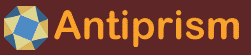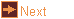# pol_recip - polar reciprocals (duals)

 Usage    |    Examples    |    Notes

## Usage

```

Usage: pol_recip [options] [input_file]

Read a file in OFF format and make a polar reciprocal from the face
planes. If input_file is not given the program reads from standard input.

Options
-h,--help this help message (run 'off_util -H help' for general help)
--version version information
-c <cent> reciprocation centre (default: C)
X,Y,Z - centre with these coordinates, 0 for origin
C - vertex centroid,    M - mid-sphere (approximate)
R - circumcentre (avg)
e - edge balanced,      E - edge balanced with inversion
v - vert/face balanced, V - vert/face balanced with inversion
a - v/f/e balanced      A - v/f/e balanced with inversion
-C <init> initial value for a centre calculation, in form 'X,Y,Z', 0 for
origin, C to use centroid (default), M to calculate approximate
mid-sphere
v - nearest vertex distance, V - furthest vertex distance
e - nearest edge distance,   E - furthest edge distance
f - nearest face distance,   F - furthest face distance
or a comma separated list of vertex indices (starting from 0)
and the distance is to the space containing those vertices
-i        transform dual by reflecting in centre point
-I <dist> maximum distance to project any normal or infinite dual vertex
(default: 1e+15), if 0 then use actual distances and delete
infinite points
-x        exclude extra elements added to duals with ideal vertices
-n <itrs> maximum number of iterations (default: 10000)
-l <lim>  minimum distance change to terminate, as negative exponent
(default: 12 giving 1e-12)
-a        append dual to original polyhedron
-o <file> write output to file (default: write to standard output)

```

## Examples

Make the dual of an octahedron, a cube.

```pol_recip oct | antiview
```

Make the dual pair of an octahedron and a cube.
```pol_recip -a oct | antiview
```

Make the dual pair of a cuboctahedron and rhombic dodecahedron, with the reciprocation radius touching the nearest faces (first command) and farthest faces (second command) causing a dual vertex to lie on faces at this distance
```pol_recip -a -r f cubo | off_color -r A0.4,f | antiview
pol_recip -a -r F cubo | off_color -r A0.4,f | antiview
```

Specify a face plane of a polyhedron by three of its index numbers, make a dual with a vertex that lies on this face plane, no matter where the reciprocation centre is
```pol_recip -a -c 0,0,0.2  -r 2,3,5 pyr5 | off_color -r A0.4,f | antiview
pol_recip -a -c 0,0,-0.4 -r 2,3,5 pyr5 | off_color -r A0.4,f | antiview
```

A canonical antihemaphrodite is self-dual, but not in the centroid (first command). The correct centre can be found as a midsphere centre (second command)
```polygon ant -s antih 4 | canonical | pol_recip -a | antiview
polygon ant -s antih 4 | canonical | pol_recip -a -c M -n 10000 | antiview
```

Pyramids have a number interesting reciprocation centres, e.g. a default "mid-centre" (first command), an external "mid-center" (second command), a self-dual "edge-balanced" centre (third command)
```polygon -l 1.5 pyr 7 | off_color -f blue | pol_recip -c M -n 50000 -a | antiview
polygon -l 1.5 pyr 7 | off_color -f blue | pol_recip -C 0,0,-1 -c M -n 50000 -a | antiview
polygon -l 1.5 pyr 7 | off_color -f blue | pol_recip -c e -n 50000 -a | antiview
```

When a polyhedron has a face plane passing through the reciprocation centre, this is dualised into a an ideal vertex at infinity. To maintain the visual symmetry of the dual, and emphasise that the vertex can be reached in two opposite directions, the vertex is placed far away (control distance with -I, and suppress this with -x). The first two commands show the dual as the reciprocation centre is above and below the base face of the triangular pyramid. Then on the plane (command 3), and again, but with the duplicated vertices surpressed (command 4)
```pol_recip -c 0,0,0.05   -r 0,1 -a pyr3 | antiview -R -90,0,0
pol_recip -c 0,0,-0.05  -r 0,1 -a pyr3 | antiview -R -90,0,0
pol_recip -c 0,0,0.00   -r 0,1 -a pyr3 | antiview -R -90,0,0
pol_recip -c 0,0,0.00  -x -r 0,1 -a pyr3 | antiview -R -90,0,0
```

## Notes

A dual of a convex polyhedron is normally made with the centre of reciprocation at the polyhedron centre and the radius to just touch the edges.

Some polyhedra have faces passing through their natural centre. This causes a problem when making a dual because the vertex which is dual to this face should be infinitely far away. pol_recip allows these vertices to be included by placing them at a specified (probably very large) distance normal to the face. Any programs dealing with these distant vertices (e.g. povray) can interpret these distant vertices accordingly.

The default reciprocation centre and radius are found by the following algorithm. It aims to find a reciprocation sphere that is balanced, in the sense that the polyhedron and its dual have the same relationship with the sphere.

```   centre = centroid of vertices of base polyhedron
radius = average distance from centre to edges
LOOP:
dual = polar reciprocal of base, using centre and radius

invert dual in centre point

edge_centroid    = centroid of the nearest points to the centre
on the base's edges and duals edges
radius_sum_base  = sum of distances from the centre to the nearest
point to the centre on the base's edges
radius_sum_dual  = sum of distances from the centre to the nearest
point to the centre on the dual's edges

if loop count is even:
centre = 0.9*centre + 0.1*edge_centroid
if loop count is odd:

finish loop if change in centre and radius are small enough

```

The aim is that this will be a similar reciprocation method. That is to say

• It will be reciprocal - it will always reciprocate a polyhedron into the same polyhedron dual, and it will always reciprocate the dual into the original polyhedron
• It will respect similarity - similar polyhedra will have similar dual-pairs and similar duals

The default method above tends to reciprocate in the midsphere, if it exists.

The other available balanced reciprocation methods use the centroid of combined face near-points and vertex offsets, or the centroid of the near-points of all three elements combined. For all three cases the dual may be inverted in the reciprocation centre before the centroid calculation.

-r e may select a sphere suitable for self-duality.

-r v and -r V may always give the same results.